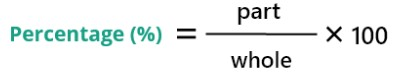# Percentage Calculator

To use percentage calculator, select percentage type, enter the input, and click the submit button

What is
% of

Give Us Feedback

## Percentage Calculator

Percentage calculator is used to find the percentage of various types. This percentage calculator uses the formula of percentage to evaluate the result.

## What is the percentage?

A relative value representing the 100th part of any quantity is said to be the percentage. Ten percent is denoted as 10% and is the 10th part of 100. Hence, 100% denotes the whole of the given quantity.

## Percentage formula## Types of percentage

There are various types of percentages used to find the 100th part of any type of quantity.

1. What is % of?
2. Is what % of?
3. Is % of what?
4. Out of what is %?
5. What is % of is?
6. % of what is?
7. % of is what?
8. Out of what is %?
9. What out of is %?

### Note

Remember how to convert percentage to decimal and decimal to percentage.

Percentage to decimal

Divide by 100 to remove the “%” sign. For example, 12% = 12/100.

Decimal to percentage

To add a percentage sign, multiply the number by 100. For example, 0.59 * 100 = 59%

## How to find percentage?

There are 9 different kinds of percentages to find the percentage or number. Follow the below examples to learn all the kinds of percentages.

1. ### What is % of?

Percentage (P%) * U = V

Example

What is 20% of 280?

Solution

Step 1: Write the given problem according to the formula.

20% * 280 = V

Step 2: Convert the percentage into a fraction by taking 100 in the denominator.

20/100 * 280 = V

0.20 * 280 = V

Step 3: Multiply both terms.

56 = V

Hence, the 20% of 280 is 56.

1. ### Is what % of?

Percentage (P%) = V/U

The result will come in decimals, you have to multiply the decimal by 100 to get the percentage form.

Example

What percent of 200 is 30?

Solution

Step 1: Write the given problem according to the formula.

Percentage (P%) = 30/200

Step 2: Convert the fraction into a decimal.

Percentage (P%) = 0.15

Step 3: Now multiply the decimal by 100 to get the percentage.

Percentage (P%) = 0.15 x 100

Percentage (P%) = 15%

1. ### Is % of what?

U = V/P%

Example

45 is 30% of what number?

Solution

Step 1: Write the given problem according to the formula.

V = 45

P% = 30

U = 45/30%

Step 2: Convert the percentage into a fraction by dividing it by 100.

U = 45/(30/100)

U = 45/0.3

Step 3: Divide the above expression.

U = 45/0.3 = 150

Hence, 45 is 30% of 150.

1. ### Out of what is %?

P% = V/U

Example

What percent of 340 is 60?

Solution

Step 1: Write it according to the formula P% = V/U.

P% = 60/340

Step 2: Divide the above term to convert the fraction into a decimal.

P% = 0.1765

Step 3: Now multiply the decimal by 100 to get the percentage.

P% = 0.1765 * 100

P% = 17.65%

Hence, 60 out of 340 is 17.65%.

1. ### What is % of is?

P% = V/U

Example

30 of what is 40%?

Step 1: Write the terms according to the formula.

P% = 40%

V = 30

U =?

P% = V/U

40% = 30/U

U = 30/40%

Step 2: Convert the percentage into a decimal.

U = 30/(40/100)

U = 30/0.4

Step 3: Divide the above expression.

U = 75

Hence, 30 of 75 is 40%.

1. ### % of what is?

U = V/P%

Example

35% of what is 12?

Solution

Step 1: Write the terms according to the formula.

P% = 35%

V = 12

U =?

P% = V/U

35% = 12/U

U = 12/35%

Step 2: Convert the percentage into a decimal.

U = 12/(35/100)

U = 12/0.35

Step 3: Divide the above expression.

U = 34.29

Hence, 35% of 34.29 is 12.

1. ### % of is what?

V = P% * U

Example

26% of 376 is what?

Solution

Step 1: Write the terms according to the formula.

P% = 26%

U = 376

V =?

V = P% * U

V = 26% * 376

Step 2: Convert the percentage into a fraction.

V = 26/100 * 376

Step 3: Convert the fraction into a decimal.

V = 0.26 * 376

Step 4: Now multiply the above expression.

V = 97.76

Hence, 26% of 376 is 97.76

1. ### What out of is %?

V = P% * U

Example

What out of 450 is 63%?

Solution

Step 1: Write the terms according to the formula.

P% = 63%

U = 450

V =?

V = P% * U

V = 63% * 450

Step 2: Convert the percentage into a fraction.

V = 63/100 * 450

Step 3: Convert the fraction into a decimal.

V = 0.63 * 450

Step 4: Now multiply the above expression.

V = 283.5

Hence, 283 out of 450 is 63%.

1. ### What is % of out of?

P% = V/U

Example

What is % of 245 out of 678?

Solution

Step 1: Write the terms according to the formula.

P% =?

U = 678

V =245

P% = V/U

P% = 245/678

Step 2: Convert the fraction into a decimal.

P% = 0.3614

Step 3: Now multiply the decimal by 100 to get the percentage.

P% = 0.3614 * 100

P% = 36.14%

Hence, 36.14% is the percent of 245 out of 678.

### Other Languages